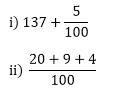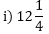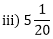×#### Thank you for registering.

One of our academic counsellors will contact you within 1 working day.

Click to Chat

1800-1023-196

+91-120-4616500

CART 0

• 0

MY CART (5)

Use Coupon: CART20 and get 20% off on all online Study Material

ITEM
DETAILS
MRP
DISCOUNT
FINAL PRICE
Total Price: Rs.

There are no items in this cart.
Continue Shopping• Complete JEE Main/Advanced Course and Test Series
• OFFERED PRICE: Rs. 15,900
• View Details

```Chapter 7: Decimals – Exercise 7.3

Question: 1

i) Five hundred twenty five and forty hundredths

ii) Twelve and thirty five thousandths

iii) Fifteen and seventeen thousandths

iv) Eighty eight and forty eight hundredths

Solution:

i) 525 + 40100 = 525.40
ii) 12 + 351000 = 12.035
iii) 15 + 171000 = 15.017
iv) 88 + 48100 = 88.48

Question: 2Solution:

i) We have 1 hundred, 3 tens, 7 ones and 5 hundredths. Therefore, the decimal is 137.05
ii) We have 2 tens, 9 ones and 4 hundredths. Therefore, the decimal is 29.04

Question: 3

i) 8/100

ii) 300/1000

iii) 18/1000

iv) 208/100

Solution:

i) We have 8 hundredths. Therefore, the decimal is 0.08
ii) In its lowest form, the fraction is 310. We have 3 tenths. Therefore, the decimal is 0.3
iii) We have eighteen thousandths. Therefore the decimal is 0.018
iv) 208100 = 200100 + 8100 = 2 + 8100

We have 2 and 8 hundredths. Therefore, the decimal is 2.08

888/1000

8881000 = 8001000 + 801000 + 81000 = 810 + 8100 + 81000

We have 8 tenths, 8 hundredths and 8 thousandths.Therefore, the decimal is 0.888

Question: 4ii) 718 = 7 + 18

iii) 5120 = 5 + 120

Solution:

i) 1214 = 12 + 14

= 12 + 254(25) = 12 + 25100 = 12.25

ii) 7 + 1(1258)(125) = 7 + 1251000 = 7.125

iii) 5 + 1(520)(5) = 5 + 510 = 5.05

Question: 5

i) 0.04iv) 1.20

v) 17.38

Solution:

i)

= 0 + 0.04

= 0 + 4 hundredths

= 0 + 4100

= 125

ii)

2.34

= 2 + 0.34

= 2 + 34 hundredths

= 2 + 34100

= 2(100100) + 34100

= 200100 + 34100

= 234100

= 11750

iii)

0.342

= 0 + 342 thousandths

= 3421000

= 171500

iv)

= 1 + 0.20

= 1 + 20 hundredths

= 1 + 20100

= 100100 + 20100

= 120100

= 65

v)

= 17 + 0.38

= 17 + 38 hundredths

= 17 + 38100

= 17(100100) + 38100

= 1700100 + 38100

= 1738100

= 86950

Question: 6

i) 2 tens, 9 ones, 4 tenths and 1 hundredths.

ii) 3 tens, we have3 tens, 4 tenths, 8 hundredths and 3 thousandths.

iii) 1 hundred, 3 tens, 7 ones and 5 hundredths.

iv) 7 tenths, 6 hundredths and 4 thousandths.

v) 2 tens, 3 ones, 2 tenths and 6 thousandths.

vi) 7 hundreds, 2 tens, 5 ones and 9 hundredths.

Solution:

i) Here, we have 2 tens, 9 ones, 4 tenths and 1 hundredths. Therefore, the decimal is 29.41

ii) Here, we have 3 tens, we have3 tens, 4 tenths, 8 hundredths and 3 thousandths. Therefore, the decimal is 30.483

iii) Here, we have 1 hundred, 3 tens, 7 ones and 5 hundredths. Therefore, the decimal is 137.05

iv) Here, we have 7 tenths, 6 hundredths and 4 thousandths . Therefore, the decimal is 0.764

v) Here, we have 2 tens, 3 ones, 2 tenths and 6 thousandths. Therefore, the decimal is 23.206

vi) Here, we have 7 hundreds, 2 tens, 5 ones and 9 hundredths. Therefore, the decimal is 725.09

```### Course Features

• 728 Video Lectures
• Revision Notes
• Previous Year Papers
• Mind Map
• Study Planner
• NCERT Solutions
• Discussion Forum
• Test paper with Video Solution